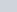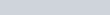# Equipotential surface : definition, types and properties.

• 2
•
•
•
•
•
•
•
2
Shares

## EQUIPOTENTIAL SURFACE

It is a self defined term, equipotential surface  means, surface which having the same electrostatic potential.

In other words it can be defined as – The surface which is the locus of all the points having same electrostatic potential is called equipotential surface.

On the equipotential surface if we move any charge particles from one point to another point then there is no work done required to move it. We can draw equipotential surfaces through any region, in which there is electric field. If all the points with same electrostatic potential in electric field are joined together then we obtained an equipotential surface.

## TYPES OF EQUIPOTENTIAL SURFACES

### EQUIPOTENTIAL POINT

If the points in an electric field are all at the same electric potential, then all the points are known as the equipotential points. The equipotential surfaces produced by a point charge or a spherically symmetrical charge is set of family of concentric circles. See fig. below.

### EQUIPOTENTIAL LINE

If all the points having equal potential in electric field (equipotential points) are connected by a line or a curve then it is called equipotential line. If this point is charge then it will be equipotential line charge. The equipotential surface of a line charge is cylindrical shapes.

### EQUIPOTENTIAL SURFACE AND VOLUME

If all the equipotential points lies on the surface then it is called equipotential surface. Further if all these equipotential points are distributed throughout the space or volume then it is called equipotential volume.

## WORK DONE IN EQUIPOTENTIAL SURFACE

We know that in any equipotential surfaces all points have same the electrostatic potential. In such surface if we try to move any charge particles from say point A to point B then for this work done in moving the charge particles is zero. Why are we saying this let’s proof it mathematically –

Let’s consider a two points A and B in any equipotential surface. The electric potential at point A isand at point B is. This both electric potential is equal to each other because it is lies on the equipotential surface.
The work done in moving of charge particle (q) from points having potentialandis given as –As we know thatandare equal then the total work done is, W = 0

## PROPERTIES OF EQUIPOTENTIAL SURFACE

These are the some properties regarding to the equipotential surfaces.

• The first property is that electric field is always perpendicular to an equipotential surface.
• Second is two equipotential surfaces can never be intersect. It is because Different equipotential surfaces have different potential. If they intersect then the point of intersection have different electrostatic potential at the same point which is not possible.
• For a point charge, the equipotential surfaces are concentric spherical shells.
• For a uniform electric field, the equipotential surfaces are planes which is normal to the x-axis.
• The direction of the equipotential surface is always from higher potential to lower potential.
• Inside a hollow charged spherical conductor the electrostatic potential is constant. This can be treated as equipotential volume. No work is required to move a charge from the centre to the surface.
• For an isolated point charge, the equipotential surface is a concentric spherical shell. Each spherical shell is a equipotential surface.
• In a uniform electric field, if you place any plane normal to the field direction is an equipotential surface.
• The spacing between equipotential surfaces helps us to identify regions of a strong and weak electric field.

1). What does equipotential mean?

Ans- Equipotential means having equal potential. If the points is of equal potential then it is called equipotential points. If this equipotential points is form line charge then it is called equipotential line. If this points are spreaded on any surface then it is called equipotential surface.

2). Can equipotential surfaces intersect?

Ans- No, equipotential surfaces can’t intersect each other. It is because different equipotential surface have different electrostatic potential if they intersect then the point of intersection have different potential at same point, which is not possible.

3). What is the angle between electric field and equipotential surface?

Ans- According to the properties of equipotential surface, electric field is always perpendicular to the equipotential surfaces. So they make angle of 90°.

4). Why do equipotential lines get further apart?

Ans- Every equipotential surface or line have a constant value of electric potential. We know that electric field is inversely proportional to the square of the position vector r. If r is large then value of electric field is smaller. More spacing between the equipotential surfaces or lines denotes the weakness of electric field as r increases.

5). What are the equipotential surfaces for an infinite line charge?

Ans – The shape of the equipotential surfaces of the infinite line charge is coaxial cylindrical.